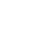Live chatDetermine whether the lines will be perpendicular when graphed.

Y = mx +c

3x - 2y = 6

-2y=-3x+6

2x + 3y = 6

-0.6667 * 1.5 = - 1.000 therefore the lines will be perpendicular since the product of their gradients is -1.

Smoking section = x,

Non – smoking = 73 + 2x

Total number of seats = 73 + 2x + x = 205

3x = 205 – 73

3x = 132

X=

Smoking section = 44 seats.

Non – smoking = 73 +44 × 2 = 161= 161 seats

## Solve the system of equations by the substitution method

x + 3y = 32 ………….. (i)

-3x + 2y = 3…………. (ii)

x = 32 – 3y …………… (iii)

## Substituting equation (iii) into equation (ii) we get

- 3(32-3y) + 2y = 3

-96 + 9y + 2y = 3

-96+11y=3

11y = 99

y = 9

Equation (iii)     x = 32 – 3y

x = 32 - 3×9

x = 5

## Solve the system of equations

x + y = 5 ……… (i)

x - y = -9 ………(ii)x = 5 – y …….. (iii)

(5 - y) – y = - 9

5 – 2y = - 9

-2y = -14

Y = 7

X = -2

A system of an equation refers to a collection of two or more linear equations which have the same set of variables. While solving a system of equations, we find the value of the unknown variables. The question below has two variables.
White = x

Red = 3x

x + 3x = 264.

4x = 264

X = 66

White should be 66 gallons while red should be 198 gallons.

0

Preparing Orders

0

Active Writers

0%

Positive Feedback

0

Support Agents

Now Accepting Apple Pay!
Limited offerget 15% off your 1st order with code first15